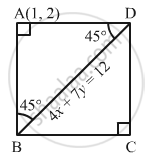Advertisement Remove all ads

# Find the Equations of the Two Straight Lines Through (1, 2) Forming Two Sides of a Square of Which 4x + 7y = 12 is One Diagonal. - Mathematics

Answer in Brief

Find the equations of the two straight lines through (1, 2) forming two sides of a square of which 4x+ 7y = 12 is one diagonal.

Advertisement Remove all ads

#### Solution

Let A (1, 2) be the vertex of square ABCD and BD be one diagonal, whose equation is 4x + 7y = 12Here, we have to find the equations of sides AB and AD, each of which makes an angle of ${45}^\circ$  with line 4x + 7y = 12

We know the equations of two lines passing through a point $\left( x_1 , y_1 \right)$ and making an angle $\alpha$ with the line whose slope is m.

$y - y_1 = \frac{m \pm \tan\alpha}{1 \mp m\tan\alpha}\left( x - x_1 \right)$
Here,

Equation of the given line is

$4x + 7y = 9$

$\Rightarrow y = - \frac{4}{7}x + \frac{9}{4}$

$\therefore x_1 = 1, y_1 = 2, \alpha = {45}^\circ , m = - \frac{4}{7}$

So, the equations of the required sides are

$y - 2 = \frac{- \frac{4}{7} + \tan {45}^\circ}{1 + \frac{4}{7}\tan {45}^\circ}\left( x - 1 \right) \text { and } y - 2 = \frac{- \frac{4}{7} - \tan {45}^\circ}{1 - \frac{4}{7}\tan {45}^\circ}\left( x - 1 \right)$

$\Rightarrow y - 2 = \frac{- \frac{4}{7} + 1}{1 + \frac{4}{7}}\left( x - 1 \right) \text { and } y - 2 = \frac{- \frac{4}{7} - 1}{1 - \frac{4}{7}}\left( x - 1 \right)$

$\Rightarrow y - 2 = \frac{3}{11}\left( x - 1 \right) \text { and } y - 2 = \frac{- 11}{3}\left( x - 1 \right)$

$\Rightarrow 3x - 11y + 19 = 0\text { and } 11x + 3y - 17 = 0$

Concept: Straight Lines - Equation of Family of Lines Passing Through the Point of Intersection of Two Lines
Is there an error in this question or solution?
Advertisement Remove all ads

#### APPEARS IN

RD Sharma Class 11 Mathematics Textbook
Chapter 23 The straight lines
Exercise 23.18 | Q 8 | Page 125
Advertisement Remove all ads
Advertisement Remove all ads
Share
Notifications

View all notifications

Forgot password?